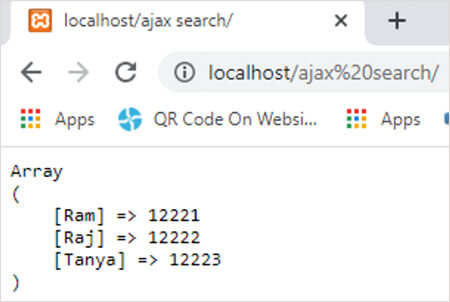array_combine function in PHP || PHP Functions

# array_combine function in php

0 1434

array_combine() function creates an array by using elements of two arrays. Items of first array are used as keys in new array and the items of second array are used as values in new array.

1. This function is used to create a new associative array with the use of two existing array.
2. The items of first array are used as keys in new array and the items of second array are used as values in new array.
3. It as an inbuilt function of PHP.

### array_combine function syntax:

`array_combine(array_1, array_2);`

Here

1. Array_1 is the array which items is used as keys.
2. Array_2 is the array which items is used as values.

### array_combine function example:

```<?php
\$array_1=array("Ram","Raj","Tanya"); // name of employees
\$array_2=array("12221","12222","12223"); // employee id of employees
\$new_array=array_combine(\$array_1,\$array_2);
echo '<pre>';
print_r(\$new_array);
?>```

Output:Share: